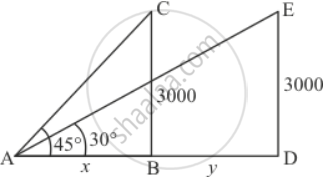Share

# The Angle of Elevation of an Aeroplane from a Point on the Ground is 45°. After a Flight of 15 Seconds, the Elevation Changes to 30°. If the Aeroplane is Flying at a Height of 3000 Metres, Find the Speed of the Aeroplane. - CBSE Class 10 - Mathematics

#### Question

The angle of elevation of an aeroplane from a point on the ground is 45°. After a flight of 15 seconds, the elevation changes to 30°. If the aeroplane is flying at a height of 3000 metres, find the speed of the aeroplane.

#### Solution

Let angle of elevation of an aeroplane is 45°. After 15 seconds angle of elevation is the change to 30°. Let DE be the height of aeroplane which is 3000 meters above the ground.

Let AB = x,BD = y, ∠CAB = 45° and ∠EAD = 30°

Here we have to find speed of aero plane.

We have the corresponding figure as followsSo we use trigonometric ratios.

In Δ ABC

=> tan A = (BC)/(AB)

=> tan 45^@ = 3000/x

=> 1 = 3000/x

=> x = 3000

=> tan A = (DE)/(AB + BD)

=> tan 30^@ = 3000/(x + y)

=> 1/sqrt3 = 3000/(3000 + y)

=> 3000 + y = 3000sqrt3

=> y = 3000sqrt3 - 3000

=> y = 3000(sqrt3 - 1)

=> y = 2196

Since 15 sec = 2196

=> sec = 2196/15 = 146.4

= (146.4 xx 3600)/1000

= 527.04

Hence the speed of aero plane is 527.04 km/h

Is there an error in this question or solution?

#### Video TutorialsVIEW ALL 

Solution The Angle of Elevation of an Aeroplane from a Point on the Ground is 45°. After a Flight of 15 Seconds, the Elevation Changes to 30°. If the Aeroplane is Flying at a Height of 3000 Metres, Find the Speed of the Aeroplane. Concept: Heights and Distances.
S